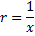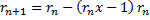# Division and Reciprocal

## By Alexander Yee

(Last updated: February 4, 2019)

Back To:

y-cruncher uses Newton's Method iteration for computing reciprocals. Division is done by multiplying by the reciprocal.

Division is needed for all the constants that y-cruncher supports except for sqrt(n) and Golden Ratio.

Algorithm

Find:Iterate:Since this is a 1st order iteration, the number of correct digits doubles with each iteration.

Like the iteration for the Inverse Square Root, this arrangement was chosen for the destructive cancellation between rn x - 1.

Implementation

Reciprocal:

In y-cruncher, the initial "guess" is obtained by simply doing a double-precision division in C++ which gives 53 bits.

Again like the Inverse Square Root, y-cruncher's implementation will choose either of two iterations:Which it chooses depends on whether rn turns out to be an under-estimate or an over-estimate of the correct answer. If it is an under-estimate, it uses the second formula to avoid negative numbers. Over-estimates are faster because subtraction by 1 is free whereas subtracting from 1 requires inversion of all the bits.

Therefore, y-cruncher's implementation will purturb the result of each iteration slightly to increase the chances that it will be an overestimate for the next iteration.

As mentioned earlier, the following operations: (depending on which iteration)

• rn x - 1
• 1 - rn x

Will exhibit destructive cancellation. The top 1/3 of the product (rn x) is either all zeros, or all ones. Therefore the FFT middle product (described here) approach can be used to reduce the convolution length by 1/3.

This has the added effect of bringing the convolution length down to the same size as the second multiply - thereby making it advantageous to reuse the forward transform for rn.

Division:

Rather than computing the full reciprocal and doing a full-precision multiply by the dividend, y-cruncher combines the multiply-by-dividend with the final iteration of the reciprocal. This approach reduces both the computational and memory complexity of the operation.

(I have since lost the reference to the paper which describes this method.)

Complexity Analysis

Complexity (Asymptotic):

Reciprocal: O( n log(n) )

Division: O( n log(n) )

The complexity of each iteration is O( M(n) ). Since Newton's Method is a self-correcting algorithm, full precision is not needed thoughout the iteration. Therefore the sum of all the iterations is a geometric sequence resulting in the same complexity: O( M(n) ).

If we assume that multiplication is M(n) = n log(n), then the entire operation is O( n log(n) ).

Complexity (Practical):

Reciprocal:

Memory: 1.67 M(n)

Swap: 1.75 M(n)

Division:

Memory: 2.167 M(n)

Swap: 2.250 M(n)

We'll omit the analysis for this, but it follows the same idea as the Inverse Square Root.

The complexities for division shown here are for floating-point approximations. If correct rounding is needed (as is the case for integer division), the cost will be higher due to the need for a multiply-back/subtract to check the result. Nevertheless y-cruncher does not (currently) need such an operation.

Overall, large number division is actually pretty fast (less than 3 multiplications). This is in stark contrast to word-sized arithmetic on hardware where division is many times slower than multiplication.

Other Algorithms

The Divide-and-Conquer algorithm for division is the other asymptotically fast algorithm. But it is more complicated and only achieves O( log(n) * M(n) ).

Nevertheless, people still use it because it doesn't require floating-point arithmetic. Using floating-point arithmetic inevitably requires dealing with rounding corner cases.